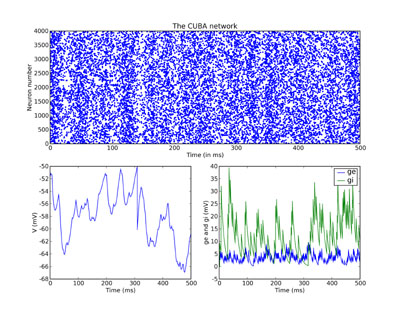# Tutorial 2c: The CUBA network¶

In this part of the tutorial, we set up our first serious network that actually does something. It implements the CUBA network, Benchmark 2 from:

Simulation of networks of spiking neurons: A review of tools and strategies (2006). Brette, Rudolph, Carnevale, Hines, Beeman, Bower, Diesmann, Goodman, Harris, Zirpe, Natschlager, Pecevski, Ermentrout, Djurfeldt, Lansner, Rochel, Vibert, Alvarez, Muller, Davison, El Boustani and Destexhe. Journal of Computational Neuroscience

This is a network of 4000 neurons, of which 3200 excitatory, and 800 inhibitory, with exponential synaptic currents. The neurons are randomly connected with probability 0.02.

```from brian import *

taum = 20 * ms          # membrane time constant
taue = 5 * ms          # excitatory synaptic time constant
taui = 10 * ms          # inhibitory synaptic time constant
Vt = -50 * mV          # spike threshold
Vr = -60 * mV          # reset value
El = -49 * mV          # resting potential
we = (60 * 0.27 / 10) * mV # excitatory synaptic weight
wi = (20 * 4.5 / 10) * mV # inhibitory synaptic weight

eqs = Equations('''
dV/dt  = (ge-gi-(V-El))/taum : volt
dge/dt = -ge/taue            : volt
dgi/dt = -gi/taui            : volt
''')
```

So far, this has been pretty similar to the previous part, the only difference is we have a couple more parameters, and we’ve added a resting potential `El` into the equation for `V`.

Now we make lots of neurons:

```G = NeuronGroup(4000, model=eqs, threshold=Vt, reset=Vr)
```

Next, we divide them into subgroups. The `subgroup()` method of a `NeuronGroup` returns a new `NeuronGroup` that can be used in exactly the same way as its parent group. At the moment, the subgrouping mechanism can only be used to create contiguous groups of neurons (so you can’t have a subgroup consisting of neurons 0-100 and also 200-300 say). We designate the first 3200 neurons as `Ge` and the second 800 as `Gi`, these will be the excitatory and inhibitory neurons.

```Ge = G.subgroup(3200) # Excitatory neurons
Gi = G.subgroup(800)  # Inhibitory neurons
```

Now we define the connections. As in the previous part of the tutorial, `ge` is the excitatory current and `gi` is the inhibitory one. `Ce` says that an excitatory neuron can synapse onto any neuron in `G`, be it excitatory or inhibitory. Similarly for inhibitory neurons. We also randomly connect `Ge` and `Gi` to the whole of `G` with probability 0.02 and the weights given in the list of parameters at the top.

```Ce = Connection(Ge, G, 'ge', sparseness=0.02, weight=we)
Ci = Connection(Gi, G, 'gi', sparseness=0.02, weight=wi)
```

Set up some monitors as usual. The line `record=0` in the `StateMonitor` declarations indicates that we only want to record the activity of neuron 0. This saves time and memory.

```M = SpikeMonitor(G)
MV = StateMonitor(G, 'V', record=0)
Mge = StateMonitor(G, 'ge', record=0)
Mgi = StateMonitor(G, 'gi', record=0)
```

And in order to start the network off in a somewhat more realistic state, we initialise the membrane potentials uniformly randomly between the reset and the threshold.

```G.V = Vr + (Vt - Vr) * rand(len(G))
```

Now we run.

```run(500 * ms)
```

And finally we plot the results. Just for fun, we do a rather more complicated plot than we’ve been doing so far, with three subplots. The upper one is the raster plot of the whole network, and the lower two are the values of `V` (on the left) and `ge` and `gi` (on the right) for the neuron we recorded from. See the PyLab documentation for an explanation of the plotting functions, but note that the `raster_plot()` keyword `newfigure=False` instructs the (Brian) function `raster_plot()` not to create a new figure (so that it can be placed as a subplot of a larger figure).

```subplot(211)
raster_plot(M, title='The CUBA network', newfigure=False)
subplot(223)
plot(MV.times / ms, MV / mV)
xlabel('Time (ms)')
ylabel('V (mV)')
subplot(224)
plot(Mge.times / ms, Mge / mV)
plot(Mgi.times / ms, Mgi / mV)
xlabel('Time (ms)')
ylabel('ge and gi (mV)')
legend(('ge', 'gi'), 'upper right')
show()
```